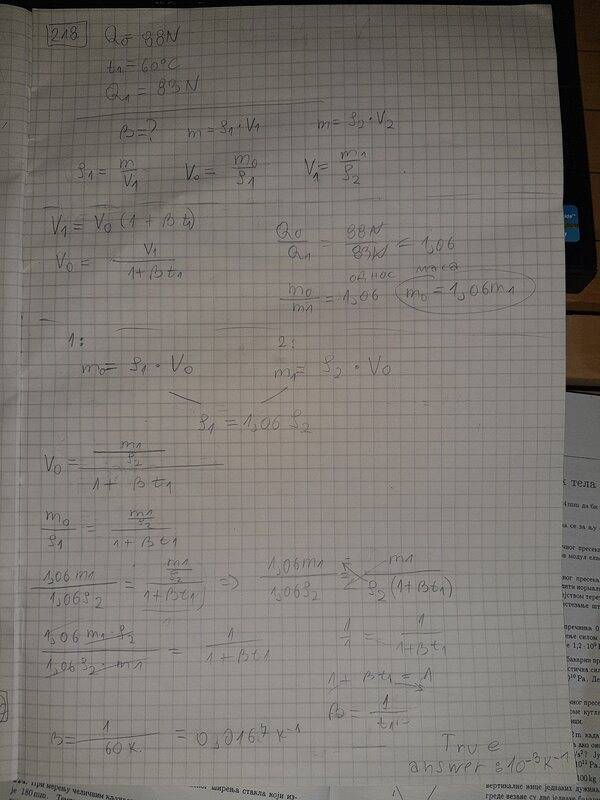# Coefficient of volumetric expansion of gasoline at different temperatures

Doshke
Homework Statement:
Task: The weight of gasoline at 0 ° C is 88N. At 60 °C the weight of the same volume of gasoline is 83N. What is the coefficient of volume expansion of gasoline?
Relevant Equations:
in the picture below are
I tried to solve the task in different ways, I had different ideas but none of them led me to the correct solution.

I would be grateful if someone would explain at least the initial part of the task to me.Homework Helper
Gold Member
2022 Award
Where does the equation ##\frac{m_0}{m_1}=1.06## come from? There is no change to the mass.

Homework Helper
Gold Member
You have the right equation relating the final volume to the initial volume, ##V_f=V_i(1+\beta \Delta T).## I think the easiest way to proceed from here is to find ##V_f## assuming constant mass. Then ##\rho_i V_i=\rho_f V_f.## Perhaps you can continue from here on your own.

•Doshke
Homework Helper
Gold Member
Where does the equation ##\frac{m_0}{m_1}=1.06## come from? There is no change to the mass.
The problem doesn't say anything to this effect, but it is instinctive to think that the gasoline is contained in a tank and some of the fluid spilling over when the temperature rises. (That was my impulsive original thought.) I have seen a similar problem in which both gasoline and tank expand and one has to find the amount of spilled gasoline.

Homework Helper
Gold Member
2022 Award
The problem doesn't say anything to this effect, but it is instinctive to think that the gasoline is contained in a tank and some of the fluid spilling over when the temperature rises. (That was my impulsive original thought.) I have seen a similar problem in which both gasoline and tank expand and one has to find the amount of spilled gasoline.
Yes, I should have been clearer.
@Doshke's algebra started by taking mass constant and computing the change in volume. That would have worked, but it then switched to taking it as a change in mass. That also would work, but unfortunately the two approaches got interwoven and cancelled, leading to the loss of the 1.06 factor.

•Doshke
Doshke
You have the right equation relating the final volume to the initial volume, ##V_f=V_i(1+\beta \Delta T).## I think the easiest way to proceed from here is to find ##V_f## assuming constant mass. Then ##\rho_i V_i=\rho_f V_f.## Perhaps you can continue from here on your own.

Thank you for your help @haruspex @kuruman I managed to do the task.
This observation saved me
##\rho_i V_i=\rho_f V_f.##

•kuruman